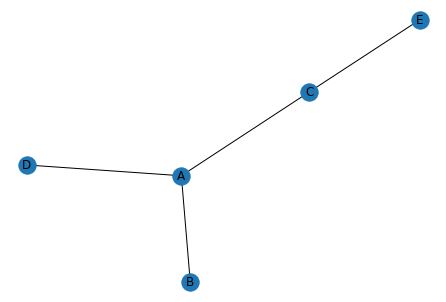This example is probably the most basic network chart you can draw.

A network chart is constituted by nodes. These nodes are interconnected by edges. So a basic format is a data frame where each line describes a connection.

Here we construct a data frame with 4 lines, describing the 4 connections of this plot! So if you have a csv file with your connections, load it and you are ready to visualise it!

Next step: customise the chart parameters!

``````# libraries
import pandas as pd
import numpy as np
import networkx as nx
import matplotlib.pyplot as plt

# Build a dataframe with 4 connections
df = pd.DataFrame({ 'from':['A', 'B', 'C','A'], 'to':['D', 'A', 'E','C']})